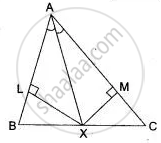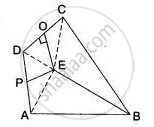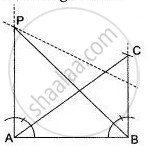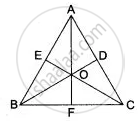# ICSE solutions for Class 10 Mathematics chapter 14 - Loci (Locus and its Constructions) [Latest edition]

#### Chapters## Chapter 14: Loci (Locus and its Constructions)

Prove the FollowingFigure Based QuestionsGraphical Depiction
Prove the Following

### ICSE solutions for Class 10 Mathematics Chapter 14 Loci (Locus and its Constructions) Prove the Following

Prove the Following | Q 1

The bisector of ∠ B and ∠C of a quadrilateral ABCD intersect in P, show that P is equidistant from the opposite sides AB and CD.

Prove the Following | Q 2

Prove that the common chord of two intersecting circles is bisected at right angles by the line of centres.

Prove the Following | Q 3

In ΔABC, the bisector AX of ∠A intersects BC ar X. XL ⊥ AB and XM ⊥ AC are drawn (Fig.) is XL = XM? why or why not?Prove the Following | Q 4

In Fig. ABCD is a quadrilateral in which AB = BC. E is the point of intersection of the right bisectors of AD and CD. Prove that BE bisects ∠ABC.Figure Based Questions

### ICSE solutions for Class 10 Mathematics Chapter 14 Loci (Locus and its Constructions) Figure Based Questions

Figure Based Questions | Q 1

Without using set squares or protractor, construct a quadrilateral ABCD in which ∠ BAD = 45° , AD = AB = 6 cm, BC= 3.6 cm and CD=5 cm. Locate the point P on BD which is equidistant from BC and CD.

Figure Based Questions | Q 2

Without using set squares or protractor construct a triangle ABC in which AB = 4 cm, BC = 5 cm and ∠ABC = 120°.
(i) Locate the point P such that ∠BAp = 90° and BP = CP.
(ii) Measure the length of BP.Figure Based Questions | Q 3

State and draw the locus of a swimmer maintaining the same distance from a lighthouse.

Figure Based Questions | Q 4

State and draw the locus of a point equidistant from two given parallel lines.

Figure Based Questions | Q 5

l is the perpendicular bisector of line segment PQ and R is a point on the same side of l as P. The segment QR intersects l at X. Prove that PX + XR = QR.

Figure Based Questions | Q 6

Construct a Δ ABC, with AB = 6 cm, AC = BC = 9 cm; find a point 4 cm from A and equidistant from B and C.

Figure Based Questions | Q 7

Given a Δ ABC with unequal sides. Find a point which is equidistant from B and C as well as from AB and AC.

Figure Based Questions | Q 8

Prove that the common chord of two intersecting circles is bisected at right angles by the line of centres.

Figure Based Questions | Q 9

Find the locus of the centre of a circle of radius r touching externally a circle of radius R.

Figure Based Questions | Q 10

ΔPBC and ΔQBC are two isosceles triangles on the same base. Show that the line PQ is bisector of BC and is perpendicular to BC.

Figure Based Questions | Q 11

Using a ruler and compass only:
(i) Construct a triangle ABC with BC = 6 cm, ∠ABC = 120° and AB = 3.5 cm.
(ii) In the above figure, draw a circle with BC as diameter. Find a point 'P' on the circumference of the circle which is equidistant from Ab and BC.
Measure ∠BCP.

Figure Based Questions | Q 12

The diagonals of a quadrilateral bisect each other at right angles. Show that the quadrilateral is a rhombus.

Figure Based Questions | Q 13

What is the locus of points which are equidistant from the given non-collinear point A, B and C? Justify your answer.

Figure Based Questions | Q 14

Find the locus of points which are equidistant from three non-collinear points.

Figure Based Questions | Q 15

Show that the locus of the centres of all circles passing through two given points A and B, is the perpendicular bisector of the line segment AB.

Figure Based Questions | Q 16

Using ruler and compasses construct:
(i) a triangle ABC in which AB = 5.5 cm, BC = 3.4 cm and CA = 4.9 cm.
(ii) the locus of point equidistant from A and C.
(iii) a circle touching AB at A and passing through C.

Figure Based Questions | Q 17

Using only a ruler and compass construct ∠ABC = 120°, where AB = BC = 5 cm.
(i) Mark two points D and E which satisfy the condition that they are equidistant from both ABA and BC.
(ii) In the above figure, join AD, DC, AE and EC. Describe the figures:
(a) AECB, (b) ABD, (c) ABE.

Figure Based Questions | Q 18

ΔPBC and ΔQBC are two isosceles triangles on the same base BC but on the opposite sides of line BC. Show that PQ bisects BC at right angles.

Figure Based Questions | Q 19

ΔPBC, ΔQBC and ΔRBC are three isosceles triangles on the same base BC. Show that P, Q and R are collinear.

Figure Based Questions | Q 20

Without using set squares or protractor construct:
(i) Triangle ABC, in which AB = 5.5 cm, BC = 3.2 cm and CA = 4.8 cm.
(ii) Draw the locus of a point which moves so that it is always 2.5 cm from B.
(iii) Draw the locus of a point which moves so that it is equidistant from the sides BC and CA.
(iv) Mark the point of intersection of the loci with the letter P and measure PC.

Figure Based Questions | Q 21

Use ruler and compasses only for this question. Draw a circle of radius 4 cm and mark two chords AB and AC of the circle of length f 6 cm and 5 cm respectively.
(i) Construct the locus of points, inside the circle, that are equidistant from A and C. Prove your construction.
(ii) Construct the locus of points, inside the circle, that are equidistant from AB and AC.

Figure Based Questions | Q 22

Draw two intersecting lines to include an angle of 30°. Use ruler and compasses to locate points which are equidistant from these Iines and also 2 cm away from their point of intersection. How many such points exist?

Figure Based Questions | Q 23

How will you find a point equidistant from three given points A, B, C which are not in the same straight line?

Figure Based Questions | Q 24

Without using set squares or protractor.
(i) Construct a ΔABC, given BC = 4 cm, angle B = 75° and CA = 6 cm.
(ii) Find the point P such that PB = PC and P is equidistant from the side BC and BA. Measure AP.

Figure Based Questions | Q 25

In Fig. AB = AC, BD and CE are the bisectors of ∠ABC and ∠ACB respectively such that BD and CE intersect each other at O. AO produced meets BC at F. Prove that AF is the right bisector of BC.Figure Based Questions | Q 26

Given: ∠BAC, a line intersects the arms of ∠BAC in P and Q. How will you locate a point on line segment PQ, which is equidistant from AB and AC? Does such a point always exist?

Figure Based Questions | Q 27

The bisectors of ∠B and ∠C of a quadrilateral ABCD intersect in P. Show that P is equidistant from the opposite sides AB and CD.

Figure Based Questions | Q 28

Use ruler and compasses only for this question. Draw a circle of radius 4 cm and mark two chords AB and AC of the circle of length f 6 cm and 5 cm respectively.
(i) Construct the locus of points, inside the circle, that are equidistant from A and C. Prove your construction.
(ii) Construct the locus of points, inside the circle, that are equidistant from AB and AC.

Figure Based Questions | Q 29

Use ruler and compasses only for the following questions:
Construct triangle BCP, when CB = 5 cm, BP = 4 cm, ∠PBC = 45°.
Complete the rectangle ABCD such that :
(i) P is equidistant from AB and BC and
(ii) P is equidistant from C and D. Measure and write down the length of AB.

Figure Based Questions | Q 30

Ruler and compass only may be used in this question. All construction lines and arcs must be clearly shown, and be of sufficient length and clarity to permit assessment.
(i) Construct Δ ABC, in which BC = 8 cm, AB = 5 cm, ∠ ABC = 60°.
(ii) Construct the locus of point inside the triangle which are equidistant from BA and BC.
(iii) Construct the locus of points inside the triangle which are equidistant from B and C.
(iv) Mark as P, the point which is equidistant from AB, BC and also equidistant from B and C.
(v) Measure and record the length of PB.

Figure Based Questions | Q 31

Ruler and compasses only may be used in this question. All construction lines and arcs must be clearly shown, and be of sufficient length and clarity to permit assessment.
(i) Construct a ΔABC, in which BC = 6 cm, AB = 9 cm and ∠ABC = 60°.
(ii) Construct the locus of the vertices of the triangles with BC as base, which are equal in area to ΔABC.
(iii) Mark the point Q, in your construction, which would make ΔQBC equal in area to ΔABC, and isosceles.
(iv) Measure and record the length of CQ.

Figure Based Questions | Q 32

Given ∠BAC (Fig), determine the locus of a point which lies in the interior of ∠BAC and equidistant from two lines AB and AC.

Graphical Depiction

### ICSE solutions for Class 10 Mathematics Chapter 14 Loci (Locus and its Constructions) Graphical Depiction

Graphical Depiction | Q 1

Use graph paper for this question. Take 2 cm = 1 unit on both the axis.
(i) Plot the points A(1,1), B(5,3) and C(2,7).
(ii) Construct the locus of points equidistant from A and B.
(iii) Construct the locus of points equidistant from AB and AC.
(iv) locate the point P such that PA = PB and P is equidistant from AB and AC.
(v) Measure and record the length PA in cm.

## Chapter 14: Loci (Locus and its Constructions)

Prove the FollowingFigure Based QuestionsGraphical Depiction## ICSE solutions for Class 10 Mathematics chapter 14 - Loci (Locus and its Constructions)

ICSE solutions for Class 10 Mathematics chapter 14 (Loci (Locus and its Constructions)) include all questions with solution and detail explanation. This will clear students doubts about any question and improve application skills while preparing for board exams. The detailed, step-by-step solutions will help you understand the concepts better and clear your confusions, if any. Shaalaa.com has the CISCE Class 10 Mathematics solutions in a manner that help students grasp basic concepts better and faster.

Further, we at Shaalaa.com provide such solutions so that students can prepare for written exams. ICSE textbook solutions can be a core help for self-study and acts as a perfect self-help guidance for students.

Concepts covered in Class 10 Mathematics chapter 14 Loci (Locus and its Constructions) are Introduction of Loci, Loci Examples, Constructions Under Loci, Theorems Based on Loci.

Using ICSE Class 10 solutions Loci (Locus and its Constructions) exercise by students are an easy way to prepare for the exams, as they involve solutions arranged chapter-wise also page wise. The questions involved in ICSE Solutions are important questions that can be asked in the final exam. Maximum students of CISCE Class 10 prefer ICSE Textbook Solutions to score more in exam.

Get the free view of chapter 14 Loci (Locus and its Constructions) Class 10 extra questions for Class 10 Mathematics and can use Shaalaa.com to keep it handy for your exam preparation